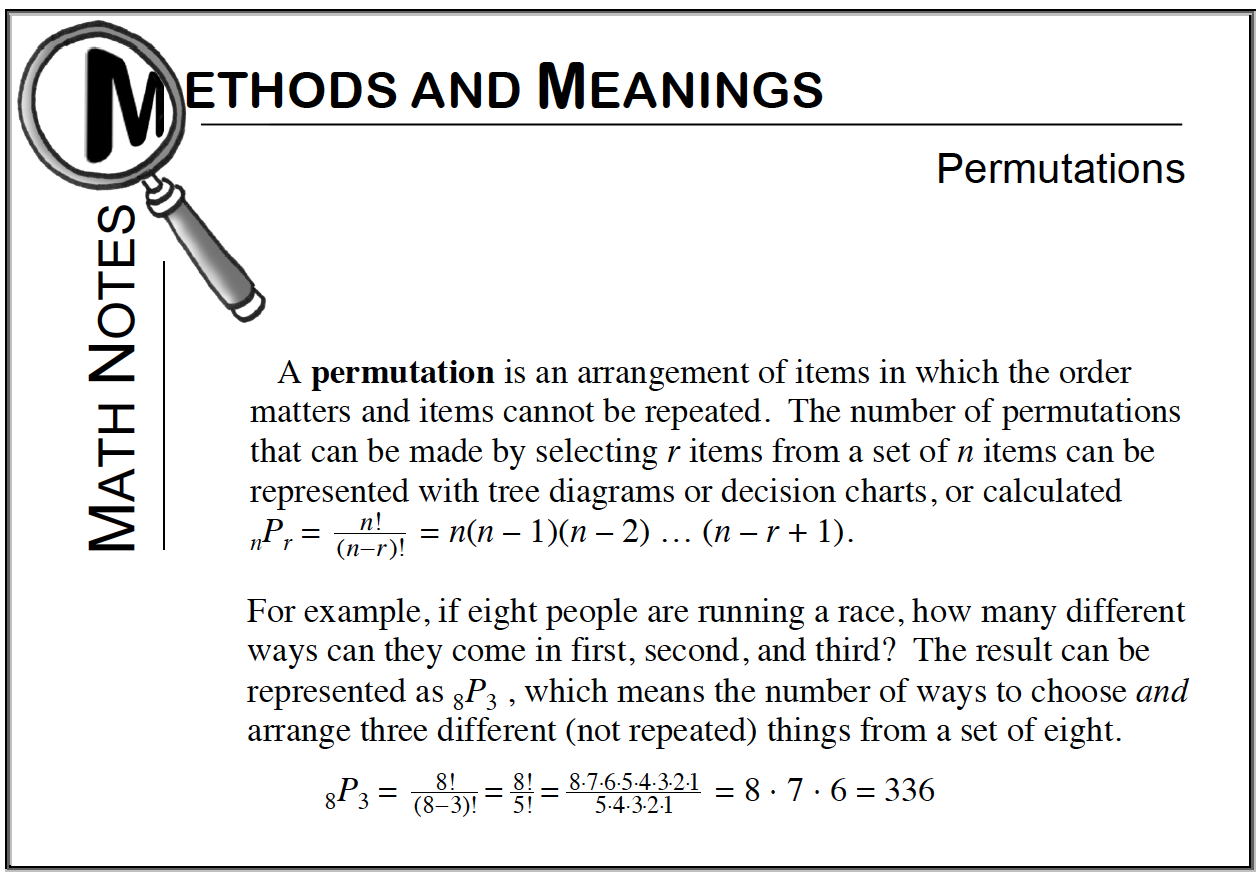### Home > CCG > Chapter 10 > Lesson 10.3.3 > Problem10-140

10-140.

What do you think $0!$ is equal to?

1. Try it on your calculator to see what you get.

$1$

2. What does $_8P_8$ mean? What should $_8P_8$ be equal to? Write $_8P_8$ using the factorial formula. Why is it necessary for $0!$ to equal $1$?

Refer to the Math Notes box below for help.

3. Do you remember how to show that $2^0 = 1$? You can use a sequence of powers of two like this: $\frac { 2 ^ { 4 } } { 2 } = 2^3$, $\frac { 2 ^ { 3 } } { 2 }= 2^2$, $\frac { 2 ^ { 2 } } { 2 }= 2^1$, so $\frac { 2 ^ { 1 } } { 2 } = 2^0$. Since $\frac { 2 ^ { 1 } } { 2 }= 1$ you also know that $2^0 = 1$.
You can construct a similar pattern for $0!$, starting with $\frac { 5 ! } { 5 }= 4!$ and then $\frac { 4 ! } { 4 }= 3!$ . Continue the pattern and make an argument to justify that $0! = 1$. Homework Help ✎

$\frac{3!}{3} - 2!$$\frac{2!}{2} = 1$$\frac{1!}{1} = 0!$

$\frac{1!}{1} = \frac{1}{1} = 1 = 0!$